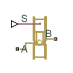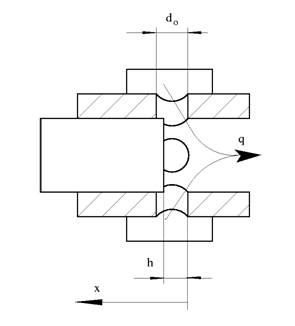# Orifice with Variable Area Round Holes

(To be removed) Hydraulic variable orifice shaped as set of round holes drilled in sleeve

The Hydraulics (Isothermal) library will be removed in a future release. Use the Isothermal Liquid library instead. (since R2020a)

## Library

Orifices

•## Description

The block models a variable orifice created by a cylindrical spool and a set of round holes drilled in the sleeve. All the holes are of the same diameter, evenly spread along the sleeve perimeter, and their center lines are located in the same plane. The flow rate through the orifice is proportional to the orifice opening and to the pressure differential across the orifice. The following schematic shows the cross section of an orifice with variable round holes, where

 q Flow rate h Orifice opening x Spool displacement from initial position d0 Orifice hole diameter### Orifice Opening Area

The aggregate opening area provided by the round holes is computed during simulation from the orifice opening, a measure of the instantaneous spool position:

where:

• h is the orifice opening. The subscript `Min` refers to the minimum value corresponding to a fully closed orifice. This value is calculated during simulation from block parameters.

• x is the spool displacement specified through physical signal port S. The subscript `0` denotes the opening offset specified in the Initial opening block parameter.

• δ is the orifice opening orientation: `+1` if positive, `-1` if negative. This value is specified in the Orifice orientation block parameter.

The minimum orifice opening hMin is calculated approximately using the expression:

`${h}_{\text{Min}}\approx \frac{{d}_{\text{o}}}{2}\left[1-\mathrm{cos}\left(\frac{\pi {D}_{\text{h,Min}}}{2{n}_{\text{o}}{d}_{\text{o}}}\right)\right]$`

where:

• do is the diameter of an individual hole. This value is obtained from the Diameter of round holes block parameter. The holes are assumed to be identical in size.

• no is the number of round holes comprising the orifice. This value is obtained from the Number of round holes block parameter.

• Dh,Min is the minimum value of the hydraulic diameter of the orifice as a whole. This value is calculated from the minimum opening area specified in the Leakage area block parameter:

`${D}_{\text{h,Min}}=\frac{\pi {d}_{\text{o}}^{2}}{4}.$`

The opening area of the orifice (the sum over the various round holes) is calculated from the conditional expression:

where:

• ALeak is the opening area in the fully closed position, when only leakage remains. This value is obtained from the Leakage area block parameter.

• AMax is the opening area in the fully open position, when the holes are open to full capacity. This value is calculated from the number and diameter of the holes:

`${A}_{\text{Max}}={n}_{\text{o}}\pi \frac{{d}_{\text{o}}^{2}}{4}$`

### Orifice Flow Rate

The net flow rate through the orifice is calculated as:

`$q={C}_{D}\cdot A\left(h\right)\sqrt{\frac{2}{\rho }}\frac{\Delta p}{{\left(\Delta {p}^{2}+{p}_{\text{Cr}}^{2}\right)}^{1/4}},$`

where:

• q is the volumetric flow rate through the orifice (the sum of the flow rates through the various holes).

• CD is the discharge coefficient of the orifice. This value is obtained from the Flow discharge coefficient block parameter.

• A is the aggregate opening area of the orifice (calculated as a function of the orifice opening h).

• Δp is the pressure differential from port A to port B:

`$\Delta p={p}_{\text{A}}-{p}_{\text{B}},$`

where p is the gauge pressure through the port indicated in the subscript.

• pCr is the calculated value of the critical pressure (from port A to port B) at which the flow is considered to shift between laminar and turbulent regimes.

Critical Pressure Differential

The calculation of the critical pressure differential varies with the setting of the Laminar transition specification block parameter:

• `By pressure ratio` — Obtain the critical pressure differential from the critical pressure ratio:

`${p}_{\text{Cr}}=\left({p}_{\text{Avg}}+{p}_{\text{Atm}}\right)\left(1-\beta \right),$`

where β is the critical pressure ratio, obtained from the Laminar flow pressure ratio block parameter, defined as the ratio of the outlet pressure to the inlet pressure at the transition point between laminar and turbulent regimes. The subscript `Atm` denotes the atmospheric value (`0.101325 MPa` at standard reference conditions) and the subscript `Avg` the average of the gauge pressures at the ports:

`${p}_{\text{Avg}}=\frac{{p}_{\text{A}}+{p}_{\text{B}}}{2},$`

• `By Reynolds number` — Obtain the critical pressure differential from the critical Reynolds number:

`${p}_{\text{Cr}}=\frac{\rho }{2}{\left(\frac{{\mathrm{Re}}_{\text{Cr}}\cdot \nu }{{C}_{D}\cdot {D}_{H}}\right)}^{2},$`

where ReCr is the critical Reynolds number, at which the flow can be said to transition between laminar and turbulent regimes, ν is the average of the kinematic viscosities at the ports, and Dh is the hydraulic diameter of the orifice:

with P as the sum of the perimeters of the holes:

`$P={n}_{\text{o}}{d}_{\text{o}}\left[2\text{acos}\left(1-\frac{2h}{{d}_{\text{o}}}\right)+2\text{asin}\left(\text{acos}\left[1-\frac{2h}{{d}_{\text{o}}}\right]\right)\right]$`

## Basic Assumptions and Limitations

• Fluid inertia is ignored.

## Parameters

Diameter of round holes

Diameter of the orifice holes. The default value is `5e-3` m.

Number of round holes

Number of holes. The default value is `6`.

Flow discharge coefficient

Semi-empirical parameter for orifice capacity characterization. Its value depends on the geometrical properties of the orifice, and usually is provided in textbooks or manufacturer data sheets. The default value is `0.6`.

Initial opening

Orifice initial opening. The parameter can be positive (underlapped orifice), negative (overlapped orifice), or equal to zero for zero lap configuration. The value of initial opening does not depend on the orifice orientation. The default value is `0`.

Orifice orientation

The parameter is introduced to specify the effect of the orifice control member motion on the valve opening. The parameter can be set to one of two options: `Opens in positive direction` or ```Opens in negative direction```. The value ```Opens in positive direction``` specifies an orifice whose control member opens the valve when it is shifted in the globally assigned positive direction. The parameter is extremely useful for building a multi-orifice valve with all the orifices being controlled by the same spool. The default value is `Opens in positive direction`.

Laminar transition specification

Select how the block transitions between the laminar and turbulent regimes:

• `Pressure ratio` — The transition from laminar to turbulent regime is smooth and depends on the value of the Laminar flow pressure ratio parameter. This method provides better simulation robustness.

• `Reynolds number` — The transition from laminar to turbulent regime is assumed to take place when the Reynolds number reaches the value specified by the Critical Reynolds number parameter.

Laminar flow pressure ratio

Pressure ratio at which the flow transitions between laminar and turbulent regimes. The default value is `0.999`. This parameter is visible only if the Laminar transition specification parameter is set to `Pressure ratio`.

Critical Reynolds number

The maximum Reynolds number for laminar flow. The value of the parameter depends on the orifice geometrical profile. You can find recommendations on the parameter value in hydraulics textbooks. The default value is `10`. This parameter is visible only if the Laminar transition specification parameter is set to `Reynolds number`.

Leakage area

The total area of possible leaks in the completely closed valve. The main purpose of the parameter is to maintain numerical integrity of the circuit by preventing a portion of the system from getting isolated after the valve is completely closed. The parameter value must be greater than 0. The default value is `1e-15` m^2.

## Global Parameters

Parameters determined by the type of working fluid:

• Fluid density

• Fluid kinematic viscosity

Use the Hydraulic Fluid block or the Custom Hydraulic Fluid block to specify the fluid properties.

## Ports

The block has the following ports:

`A`

Hydraulic conserving port associated with the orifice inlet.

`B`

Hydraulic conserving port associated with the orifice outlet.

`S`

Physical signal port to control spool displacement.

The flow rate is positive if fluid flows from port `A` to port `B`. Positive signal at the physical signal port `S` opens or closes the orifice depending on the value of the parameter Orifice orientation.

## Version History

Introduced in R2006a

expand all